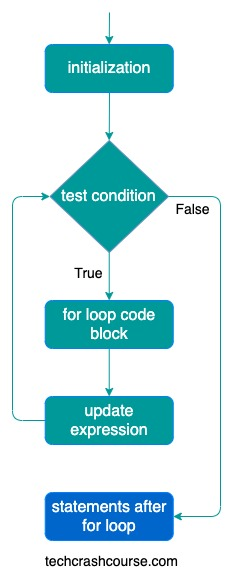# Java For Loop

For loop in java is used to execute a block of statements multiple times till the time a condition is true. The for statement provides a compact way to iterate over a range of values.

For loop is recommended by programmers if you know how many times you want to iterate.

## Simple For Loop in Java

Here is the syntax of simple for loop

```for(initialization; test_condition; update_statement) {
/* for loop code block */
statements;
}
```

For loop starts with the keyword for followed by three statements separated by semicolon and enclosed in parentheses.

• The initialization statement will be executed first and only once. We can declare and initialize any number of loop control variables here.

• Next, test_condition expression is evaluated. If the value of test_condition is true then code block of for loop will be executed otherwise the loop will terminated.

• After execution of the code block of for loop, control goes to update_statement of the loop statement which updates the loop control variables.

• test_condition expression is evaluated again. For loop iteration will continue unless test_condition expression evaluates to false.

Here is the flowchart of for loop execution### Java For Loop Example Program

```public class ForLoop {
public static void main(String[] args) {
int count;
for(count = 0; count < 5; count++){
System.out.println(count);
}
}
}
```
Output
```0
1
2
3
4
```

In above program, for loop executes print statement 5 times, till the time the value of count variable is less than 5. After each iteration the value of count variable is incremented. As soon as value of count becomes 5, for loop terminates.

Here are the execution steps for each iteration of for loop in above program.

Iteration Count Variable Test Condition
(count < 5)
For Loop
Code Block
Update Statement
1 count = 0 true Print count(0). count is incremented to 1
2 count = 1 true Print count(1). count is incremented to 2
3 count = 2 true Print count(2). count is incremented to 3
4 count = 3 true Print count(3). count is incremented to 4
5 count = 4 true Print count(4). count is incremented to 5
6 count = 5 false Not executed Not executed